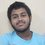# Relations

Today, my math teacher took an exam on Relations and Functions and there was this question (pretty easy one though).

Q: Prove that the relation $R$ on the set $\mathbb{N}\times \mathbb{N}$ defined by $(a,b)R(c,d)\Leftrightarrow a+d=b+c$ for all $(a,b),(c,d)\in \mathbb{N}\times \mathbb{N}$ is an equivalence relation.

Solution:

The solution that I gave was as follows,

Test for Reflexivity:

Let $(a,b)\in \mathbb{N}\times \mathbb{N}\\ \implies a+b=a+b\\ \implies a+b=b+a\\ \implies (a,b)R(a,b)~\forall (a,b)\in \mathbb{N}\times \mathbb{N} \implies R\textrm{ is reflexive. }\ldots (i)$

Test for Symmetry:

Let $(a,b),(c,d)\in \mathbb{N}\times \mathbb{N}~\land~(a,b)R(c,d)\\ \implies a+d=b+c\\ \implies c+b=d+a\\ \implies (c,d)R(a,b)~\forall (a,b),(c,d)\in \mathbb{N}\times \mathbb{N}\implies R\textrm{ is symmetric. }\ldots (ii)$

Test for Transitivity:

Let $(a,b),(c,d),(e,f)\in \mathbb{N}\times \mathbb{N}~\land~(a,b)R(c,d)~,~(c,d)R(e,f)$

$\therefore a+d=b+c~\land~c+f=d+e\\ \implies a+d=b+c\ldots (1)~\land~d+e=c+f\ldots (2)$

Subtracting $(2)$ from $(1)$, we get,

$a-e=b-f \\ \implies a+f=e+b\\ \implies (a,b)R(e,f)~\forall (a,b),(c,d),(e,f)\in \mathbb{N}\times \mathbb{N}\\ \implies R\textrm{ is transitive. }\ldots (iii)$

From $(i),(ii)$ and $(iii)$, we conclude that $R$ is an equivalence relation on $\mathbb{N}\times \mathbb{N}$

Now, my question is, does this proof contain any errors?

My teacher says that the test for transitivity is wrong because we cannot introduce $(-e)$ or $(-f)$ anywhere as they $\notin \mathbb{N}$ and as such, we cannot manipulate the equations in any way that would result in getting any non-natural integer along the steps. Instead, he showed me that the correct way will be to add both the equations instead of subtracting them and then cancelling values from both sides like this:

$a+d+c+f=b+c+d+e\implies a+f=b+e\implies (a,b)R(e,f)$

And then the proof follows the same as I did.

But the fact that he already said we cannot show the existence of non-natural integers while writing the proof self contradicts what he himself did.

When we cancel a value (say $x$) from both sides, all we do is subtract $x$ from both sides. We can also say that we add $(-x)$ on both sides.

So, when he cancelled out $d,c$ from both sides, all he did was add $(-d),(-c)$ on both sides but that contradicts his original statement that we cannot introduce any non-natural values in the proof.

So, I'm requesting all Brilliantians to help me out with this. Was there any mistake in my original solution?Note by Prasun Biswas
4 years, 10 months ago

This discussion board is a place to discuss our Daily Challenges and the math and science related to those challenges. Explanations are more than just a solution — they should explain the steps and thinking strategies that you used to obtain the solution. Comments should further the discussion of math and science.

When posting on Brilliant:

• Use the emojis to react to an explanation, whether you're congratulating a job well done , or just really confused .
• Ask specific questions about the challenge or the steps in somebody's explanation. Well-posed questions can add a lot to the discussion, but posting "I don't understand!" doesn't help anyone.
• Try to contribute something new to the discussion, whether it is an extension, generalization or other idea related to the challenge.

MarkdownAppears as
*italics* or _italics_ italics
**bold** or __bold__ bold
- bulleted- list
• bulleted
• list
1. numbered2. list
1. numbered
2. list
Note: you must add a full line of space before and after lists for them to show up correctly
paragraph 1paragraph 2

paragraph 1

paragraph 2

[example link](https://brilliant.org)example link
> This is a quote
This is a quote
    # I indented these lines
# 4 spaces, and now they show
# up as a code block.

print "hello world"
# I indented these lines
# 4 spaces, and now they show
# up as a code block.

print "hello world"
MathAppears as
Remember to wrap math in $$ ... $$ or $ ... $ to ensure proper formatting.
2 \times 3 $2 \times 3$
2^{34} $2^{34}$
a_{i-1} $a_{i-1}$
\frac{2}{3} $\frac{2}{3}$
\sqrt{2} $\sqrt{2}$
\sum_{i=1}^3 $\sum_{i=1}^3$
\sin \theta $\sin \theta$
\boxed{123} $\boxed{123}$

Sort by:

Can anybody solve the Maharashtra rmo 2008 problem 5

- 4 years, 2 months ago

Since when did school maths teachers know the basics of maths anyways? There's a reason why he's a school teacher, not a mathematics researcher. Your proof is completely correct.

Just FYI, I've been in even more ridiculous situations, the most recent of them being this-- I was given to prove the following statement in class: "show that if $x$ is a real number $>5$, then $x^2>25$ using the method of contradiction". As if using the "method of contradiction" to prove this trivial fact weren't artificial enough, I had to write all the statement formally, sort of like "let $P(x)$ be the proposition that $x>5$...". Of course, I couldn't do this and around 30 minutes of lecture followed.

- 4 years, 9 months ago

Well, proving the fact that $x\in \mathbb{R}~\land~x\gt 5\implies x^2\gt 25$ isn't so simple. It requires a complex thought process and one must have a proper knowledge of higher mathematics. I have heard that even some IMO gold medalists and top mathematical researchers call this problem as "The hardest unsolved problem in mathematics till now"! :vImage

- 4 years, 9 months ago

@Calvin Lin @Mursalin Habib @Sreejato Bhattacharya Need help here, guys!

- 4 years, 10 months ago

No problem at all!

$a + d = b + c$

$\implies a - b = c - d$

And (-b) or (-d) are negative integers.

- 4 years, 10 months ago

Yes, I think the same but my teacher doesn't. And he just scratched off my answer and gave me a lecture about logic for 30 minutes. Now, the problem that I have to face is showing the test paper to my dad. He won't even see what I wrote. He'll just say, "Y U NO BRING GOOD MARKS?" -_-

- 4 years, 10 months ago